INTERVAL ARITHMETIC

version 1.13
by Dmitry A. Kazakov

(mailbox@dmitry-kazakov.de)This library is free software; you can redistribute it and/or modify it under the terms of the GNU General Public License as published by the Free Software Foundation; either version 2 of the License, or (at your option) any later version. This library is distributed in the hope that it will be useful, but WITHOUT ANY WARRANTY; without even the implied warranty of MERCHANTABILITY or FITNESS FOR A PARTICULAR PURPOSE. See the GNU General Public License for more details. You should have received a copy of the GNU General Public License along with this library; if not, write to the Free Software Foundation, Inc., 59 Temple Place - Suite 330, Boston, MA 02111-1307, USA.

As a special exception, if other files instantiate generics from this unit, or you link this unit with other files to produce an executable, this unit does not by itself cause the resulting executable to be covered by the GNU General Public License. This exception does not however invalidate any other reasons why the executable file might be covered by the GNU Public License.

Related on-line resources:

 ARM Intel Download Interval Arithmetic for Ada Platform: v7 64- 32bit Fedora packages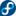precompiled and packaged using RPM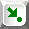CentOS packagesprecompiled and packaged using RPMDebian packages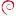precompiled and packaged for dpkgUbuntu packages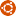precompiled and packaged for dpkgSource distribution (any platform) intervals_1_13.tgz (tar + gzip, Windows users may use WinZip)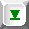Interval computations provide an alternative to traditional numeric computations. The fundamental difference is that interval computations trade result precision for accuracy, while the traditional floating-point computations do accuracy for precision. That is - the result of a traditional floating-point operation has the precision of arguments. The rounding errors are accumulated as accuracy loss. Differently to this the result of an interval operation suffers no accuracy loss, in the sense that the mathematical  outcome of the operation is contained by the result interval. Rounding errors in interval operations are accumulated as the interval width, i.e. as precision loss.

This fundamental property of interval operations yields the extension principle by which numeric operations are extended to the interval ones:

Let f be a numeric operation of N arguments: f(x1, x2, .., xN). Then the corresponding interval operation F(I1, I2, .., IN) is defined as

F(I1, I2, .., IN)=[l, u],  where

 l = Inf (f(y1, y2, .., yN)) u = Sup (f(y1, y2, .., yN)) yi∈Ii, i=1..N yi∈Ii, i=1..N

For example consider the operation +. I1=[ab], I2=[cd]. + is monotonically ascending, thus Inf is a+c and Sup is b+d and so [ab]+[cd]=[a+cb+d].

Any combination of interval operations maintain the property, but changing order of operations or splitting operations into smaller ones may change the precision of the result (the result interval width). E.g. x2 is not necessarily equal to x*x, still both contain the exact outcome.

## 1. Types and operations

The packages define a logical and interval types. The interval types are obtained through instantiation of the corresponding generic packages. Interval and numeric types can be mixed where the result has mathematical sense.

### 1.1. Tri-state logic

The package Intervals serves as a root package. It also provides tri-state logic necessary to define relational operations on intervals. The type Logical is defined as:

type Logical is (False, True, Uncertain);

The following operations are defined for Logical:

function "not" (Left : Logical) return Logical;
function "and" (Left, Right : Logical) return Logical;
function "and" (Left : Logical; Right : Boolean)
return Logical;
function "and" (Left : Boolean; Right : Logical)
return Logical;
function "or" (Left, Right : Logical) return Logical;
function "or" (Left : Logical; Right : Boolean)
return Logical;
function "or" (Left : Boolean; Right : Logical)
return Logical;
function "xor" (Left, Right : Logical) return Logical;
function "xor" (Left : Logical; Right : Boolean)
return Logical;
function "xor" (Left : Boolean; Right : Logical)
return Logical;

The following table summarizes the behaviour of three-state logical operations:

 x y x and y (&) x or y (∨) x xor y false false false false false false true false true true false uncertain false uncertain uncertain true true true true false true uncertain uncertain true uncertain uncertain uncertain uncertain uncertain uncertain

The following tables describe the operations in the form customary for the papers on logic (uncertainty is denoted as ┴):

 and 0 1 ┴ or 0 1 ┴ xor 0 1 ┴ not 0 0 0 0 0 0 1 ┴ 0 0 1 0 0 1 1 0 1 ┴ 1 1 1 1 1 1 0 ┴ 1 0 ┴ 0 ┴ ┴ ┴ ┴ 1 ┴ ┴ 0 ┴ ┴ ┴ ┴

function To_Logical (Left : Boolean) return Logical;

This function converts the argument to the corresponding fuzzy logical value.

### 1.2. Interval arithmetic

Two generic child packages Integers and Floats provide interval arithmetic for integer and floating-point types:

generic
type
Number is range <>;
package Intervals.Integers is
...
generic
type
Number is digits <>;
package Intervals.Floats is
...

The formal parameter Number is the type used for the bounds. For Intervals.Integers it is an integer type. For Intervals.Floats it is a floating-point type. The type Interval is defined by the packages as:

type Interval is record

From : Number;
To   : Number;
end record;

The conventional numeric operations are defined on intervals. Among two operands one is allowed to be of the type Number.:

function "abs" (Left : Interval) return Interval;
function
"+"   (Left : Interval) return Interval;
function "-"   (Left : Interval) return Interval;
function "**"  (Left : Interval; Right : Natural) return Interval;

function "+" (Left, Right : Interval) return Interval;
function
"+" (Left : Interval; Right : Number) return Interval;
function
"+" (Left : Number; Right : Interval) return Interval;

function
"-" (Left, Right : Interval) return Interval;
function
"-" (Left : Interval; Right : Number) return Interval;
function
"-" (Left : Number; Right : Interval) return Interval;

function
"*" (Left, Right : Interval) return Interval;
function
"*" (Left : Interval; Right : Number) return Interval;
function
"*" (Left : Number; Right : Interval) return Interval;

function
"/" (Left, Right : Interval) return Interval;
function
"/" (Left : Interval; Right : Number) return Interval;
function
"/" (Left : Number; Right : Interval) return Interval;

The interval operations over arguments [a, b] and [c, d] are defined as follows:

 [a, b] + [c, d] = [a+c, b+d] [a, b] - [c, d] = [a-d, b-c] [a, b] · [c, d] = [min (a·c, a·d, b·c, b·d), max (a·c, a·d, b·c, b·d)] [a, b] / [c, d] = [a, b] · [1/d, 1/c], if [c, d] does not contain 0

Exponentiation by a natural power [a, b]k:

 if 0∈[a, b], then Observe also that for intervals only [a, b]2⊆[a, b]·[a, b] is true. For example [-1, 2]·[-1, 2]=[-2, 4], but [-1, 2]2=[0, 4]. In general case an independency analysis of the variables involved in an interval expression might be required to improve accuracy of multiplicative operations. [a, b] 0 = [0, 1] [a, b] 2n = [0, max (a2n, b2n)] [a, b] 2n+1 = [a2n+1, b2n+1] if [a, b]>0, then [a, b] 0 = [1, 1] [a, b] k>0 = [ak, bk] if [a, b]<0, then [a, b] 0 = [1, 1] [a, b] 2n = [b2n, a2n] [a, b] 2n+1 = [a2n+1, b2n+1]

For all operations over interval bounds no overflow checks are made explicitly. Constraint_Error is propagated only when a numeric operation on bounds raises it. That depends on the behavior of the type Number:

• If Number is an integer type, overflows cause Constraint_Error, except the cases when this is explicitly suppressed by a compiler pragma or a switch;
• If Number is a floating-point type, then the value of the attribute Number'Machine_Overflows indicates if Constraint_Error will propagate. See G.2.1. Ada Reference Manual.

The result of any inexact operation (an interval) is defined to contain the exact mathematical result except the cases of overflows. For integer arithmetic, in which only division is not precise, it means that for instance, [1, 1]/2 = [0, 1], while 1/2 is 0. Division to intervals containing zero raises Constraint_Error. Floating-point arithmetic emulates ↑ and ↓ rounding. A discussion concerning implementation of interval arithmetic can be found in Introduction to interval computation, G.Alefeld, J.Herzberger; Academic Press, 1983.

The relational operations defined on intervals are:

function ">" (Left, Right : Interval) return Logical;
function
">" (Left : Interval; Right : Number) return Logical;
function
">" (Left : Number; Right : Interval) return Logical;

function
">=" (Left, Right : Interval) return Logical;
function
">=" (Left : Interval; Right : Number) return Logical;
function
">=" (Left : Number; Right : Interval) return Logical;

function
"<=" (Left, Right : Interval) return Logical;
function
"<=" (Left : Interval; Right : Number) return Logical;
function
"<=" (Left : Number; Right : Interval) return Logical;

function
"<" (Left, Right : Interval) return Logical;
function
"<" (Left : Interval; Right : Number) return Logical;
function
"<" (Left : Number; Right : Interval) return Logical;

Among two operands one is allowed to be of the type Number. Equality "=" (and so inequality "/=") is implemented as in the set theory and so the predefined equality is used. An alternative definition ([ab]=[cd] ⇔ ∀x∈[ab] ∀y∈[cd] x=y) would be rather useless. However other relational operators are implemented in exactly this sense, i.e. if @ is a relational operator defined on numbers, then:

x∈[a, b] ∀y∈[c, d] x@y ⇒ [a, b]@[c, d];

x∈[a, b] ∀y∈[c, d] x@y[a, b]@[c, d]

Otherwise it is uncertain. Thus the result of relational operations is of the type Logical.

function "&" (Left, Right : Interval) return Boolean;
function
"&" (Left : Interval; Right : Number) return Boolean;
function
"&" (Left : Number; Right : Interval) return Boolean;

The function "&" returns true if the intersection of Left and Right is not empty. Other operations defined on intervals:

function Distance (Left, Right : Interval) return Number;
function
Distance (Left : Interval; Right : Number) return Number;
function
Distance (Left : Number; Right : Interval) return Number;

This function returns the interval distance defined as max (|a-c|, |b-d|). An upper bound of the precise distance is returned.

function From (Left : Interval) return Number;
function
To   (Left : Interval) return Number;

These functions return the interval bounds.

function Is_In (Left, Right : Interval) return Boolean;
function
Is_In (Left : Number; Right : Interval) return Boolean;

These functions Is_In implement membership test. They return true if Left is a subinterval or else an element of Right.

function Is_Negative (Left : Interval) return Boolean;
function
Is_Positive (Left : Interval) return Boolean;

These functions test if the argument is negative or positive. Note that non-positive interval is not necessarily a negative one. If both functions return false, then the interval contains zero.

function Length (Left : Interval) return Number;

The interval length is defined as b-a. This function returns an upper bound of the precise result.

function To_Interval (Left : Number) return Interval;
function To_Interval (Left; Right : Number) return Interval;

These functions are used to convert numbers to intervals. The second function propagates Constraint_Error when Left is greater than Right.

## 2. Dimensioned intervals

The generic child package Measures provides an implementation of dimensioned intervals:

generic
with package
Float_Intervals is new Intervals.Floats (<>);
use
Float_Intervals;
with package Float_Measures is new Standard.Measures (Number);
package
Intervals.Measures is
...

The formal parameters are:

The type Interval_Measure is defined by the package to represent dimensioned intervals:

type Interval_Measure (SI : Unit := Units.Base.Unitless) is record
From   : Number;
To     : Number;
Offset : Number'Base;
end record;

The discriminant SI and the field Offset determine the dimension of the interval which bounds are specified by the fields From and To. The numerical values of the bounds in SI units are obtained as From + Offset and To + Offset correspondingly. For further information see the description of the package Measures.

The result of all arithmetic operations and conversions is defined to contain the precise value except for the case of an overflow while evaluation of an interval bound. Depending on the underlying floating-point type and compiler options that may or not result in Constraint_Error exception.

### 2.1. Arithmetic

The following arithmetic operations are defined on dimensioned intervals:

function "abs" (Left : Interval_Measure) return Interval_Measure;
function "+"   (Left : Interval_Measure) return Interval_Measure;
function "-"   (Left : Interval_Measure) return Interval_Measure;
function "**"  (Left : Interval_Measure; Right : Number) return Interval_Measure;

function
"+" (Left, Right : Interval_Measure) return Interval_Measure;
function "+" (Left : Interval_Measure; Right : Measure) return Interval_Measure;
function "+" (Left : Measure; Right : Interval_Measure) return Interval_Measure;

function "-" (Left, Right : Interval_Measure) return Interval_Measure;
function "-" (Left : Interval_Measure; Right : Measure) return Interval_Measure;
function "-" (Left : Measure; Right : Interval_Measure) return Interval_Measure;

function "*" (Left, Right : Interval_Measure) return Interval_Measure;
function "*" (Left : Interval_Measure; Right : Measure ) return Interval_Measure;
function "*" (Left : Interval_Measure; Right : Interval) return Interval_Measure;
function "*" (Left : Interval_Measure; Right : Number  ) return Interval_Measure;
function "*" (Left : Measure;  Right : Interval_Measure) return Interval_Measure;
function "*" (Left : Interval; Right : Interval_Measure) return Interval_Measure;
function "*" (Left : Number;  Right  : Interval_Measure) return Interval_Measure;
function "*" (Left : Interval; Right : Measure ) return Interval_Measure;
function "*" (Left : Measure;  Right : Interval) return Interval_Measure;

function "/" (Left, Right : Interval_Measure) return Interval_Measure;
function "/" (Left : Interval_Measure; Right : Interval) return Interval_Measure;
function "/" (Left : Interval_Measure; Right : Measure ) return Interval_Measure;
function "/" (Left : Interval_Measure; Right : Number  ) return Interval_Measure;
function "/" (Left : Measure;  Right : Interval_Measure) return Interval_Measure;
function "/" (Left : Interval; Right : Interval_Measure) return Interval_Measure;
function "/" (Left : Number;   Right : Interval_Measure) return Interval_Measure;

Binary additive operations "+" and "-" accept as one of the arguments a dimensioned number of the type Float_Measures.Measure. Multiplicative binary operations "*" and "/" accept as one of the arguments:

Additionally "*" and "/" are defined for a dimensioned number and a plain interval. The exception Unit_Error (defined in Units) is propagated on all unit errors as described in Measures. Constraint_Error is propagated out of multiplicative operations "*", "/" and exponentiation "**" when the dimension of the result cannot be represented because of a base unit power overflow.

function ">" (Left, Right : Interval_Measure) return Logical;
function ">" (Left : Interval_Measure; Right : Measure) return Logical;
function ">" (Left : Measure; Right : Interval_Measure) return Logical;

function ">=" (Left, Right : Interval_Measure) return Logical;
function ">=" (Left : Interval_Measure; Right : Measure) return Logical;
function ">=" (Left : Measure; Right : Interval_Measure) return Logical;

function "<=" (Left, Right : Interval_Measure) return Logical;
function "<=" (Left : Interval_Measure; Right : Measure) return Logical;
function "<=" (Left : Measure; Right : Interval_Measure) return Logical;

function "<" (Left, Right : Interval_Measure) return Logical;
function "<" (Left : Interval_Measure; Right : Measure) return Logical;
function "<" (Left : Measure; Right : Interval_Measure) return Logical;

function "&" (Left, Right : Interval_Measure) return Boolean;

In these relational operations one of the arguments can be a dimensioned value of the type Float_Measures.Measure. Differently shifted arguments are allowed, Uint_Error is propagated only if the arguments have incomparable dimensions. The function "&" returns true if the intersection of Left and Right is not empty. It is optimistic in the sense that it yields true always when the precise does. Thus computation errors may lead to a false positive, but never to a false negative.

Equality "=" (and so inequality "/=") is defined as identity. Uint_Error is not propagated out of them.

### 2.2. Operations

function Distance (Left, Right : Interval_Measure) return Measure;
function Distance (Left : Measure; Right : Interval_Measure) return Measure;
function Distance (Left : Interval_Measure; Right : Measure) return Measure;

This function returns the interval distance defined as max (|a-c|, |b-d|). An upper bound of the precise distance is returned. One of the arguments can be a dimensioned value of the type Float_Measures.Measure. Unit_Error is propagated when the arguments cannot be added because units are incompatible or differently shifted.

function From (Left : Interval_Measure) return Measure;
function
To   (Left : Interval_Measure) return Measure;

These functions return the interval bounds as dimensioned values.

function Get_Unit (Value : Interval_Measure) return Unit;

This function returns the SI measurement unit of the argument.

function Is_In (Left, Right : Interval_Measure) return Boolean;
function
Is_In (Left : Measure; Right : Interval_Measure) return Boolean;

These functions return true if Left is a subinterval or else an element of Right. One of the parameters can be a dimensioned value of the type Float_Measures.Measure. Differently shifted parameters are allowed. If Left and Right have different units, the result false. The implementation is pessimistic in the sense that the result is true only if the precise result is. It means that due to computation errors the result can be false negative, but never false positive.

function Is_Negative (Left : Interval_Measure) return Boolean;
function
Is_Positive (Left : Interval_Measure) return Boolean;

These function test if the argument is negative or positive. Note that non-positive interval is not necessarily a negative one. If both functions return false, then the interval contains zero.

function Length (Left : Interval_Measure) return Measure;

The interval length is defined as b-a. This function returns an upper bound of the precise result.

### 2.3. Conversions

function Convert (Value : Interval_Measure; Scale : Measure)
return Interval_Measure;

This function is used to convert the interval measure Value to the measurement units specified by the parameter Scale. When offsets of Value and Scale are same this is null operation. Unit_Error is propagated when conversion is impossible because units of Value and Scale are incompatible.

function Get_Value (Value : Interval_Measure) return Interval;

This function returns SI equivalent of its argument. The result is a plain interval of the type Float_Intervals.Interval.

function Get_Value_As (Value : Interval_Measure; Scale : Measure)
return Interval;

This function returns Scale equivalent of its argument. The result is a plain interval of the type Float_Intervals.Interval. Unit_Error is propagated when conversion is impossible because units of Value and Scale are incompatible.

function Normalize (Value : Interval_Measure) return Interval_Measure;

This function returns unshifted equivalent of its argument. For example when applied to an interval of Celsius degrees the result will be an equivalent interval in Kelvin.

function Shift (Value : Interval_Measure; Shift : Number'Base)
return Interval_Measure;

This function returns an equivalent of its argument  The offset of the result is determined by the parameter Shift.

function To_Interval_Measure (Left, Right : Measure) return Interval_Measure;
function To_Interval_Measure (Left, Right : Number)  return Interval_Measure;
function To_Interval_Measure (Left : Measure ) return Interval_Measure;
function To_Interval_Measure (Left : Number  ) return Interval_Measure;
function To_Interval_Measure (Left : Interval) return Interval_Measure;

These functions are used for interval composition. A pair of dimensioned or plain numbers can be used to create a dimensioned interval with the corresponding bounds. Unit_Error is propagated when the bound have incompatible units or are differently shifted. The result is dimensionless if the arguments are numeric. The variants with one argument construct a zero length interval when the argument is a number or a dimensioned number. When the argument is a plain interval, then the result is a dimensionless one.

## 3. Packages

### 3.1. Source packages

 Package Provides Intervals The type Logical and operations on it Floats Floating-point  interval arithmetic. This is a generic package. The parameter is a floating-point type Integers Integer interval arithmetic. This is a generic package. The parameter is an integer type Measures Dimensioned intervals. Float_Intervals An instantiation of Intervals.Floats with Float as the actual parameter Float_Interval_Measures An instantiation of Intervals.Measures for the type Float Integer_Intervals An instantiation of Intervals.Integers with Integer as the actual parameter

### 3.2. Related  packages

The following packages are described in the separate documents:

## 4. Installation

The software does not require special installation. The archive's content can be put in a directory and used as-is. For users of GNAT compiler the software provides gpr project files, which can be used in the Gnat Programming Studio (GPS).

For CentOS, Debian, Fedora, Ubuntu Linux distributions there are pre-compiled packages, see the links on the top of the page.

To ease use of the software with GPS, it can be integrated into the GPS using the GPS Library Installer (gps_installer). Start the gps_installer as root (or with the corresponding administrative rights to the GNAT installation directory) specifying the source directory as the argument. Follow the instructions.

 Project files Provides Use in custom project intervals Interval Arithmetic for Ada with "intervals.gpr";

## 5. Changes log

The following versions were tested with the compilers:

• GNAT Community 2018 (20180523-73)
• GNAT 8

Changes (5 Aug 2018) to the version 1.12:

• Switched to GNAT Community 2018.

The following versions were tested with the compilers:

• GNAT GPL 2015 (20150428-49)
• GNAT 4.9.2

Changes (2 April 2015) to the version 1.11:

• ARMv7 (AKA armhf) support.

Changes (1 June 2014) to the version 1.10:

• Compiled with GNAT 4.9.

The following versions were tested with the compilers:

• GNAT Pro 6.4.2 (20110614-45)
• GNAT 4.6.2 (20111027)

Changes to the version 1.9.

• Fedora and Debian packages are provided for both 32- and 64-bit architectures.

The following versions were tested with the compilers:

• GNAT Pro 6.3.1 (20100111-43)
• GNAT GPL 2009 (20090511)

Changes to the version 1.7.

• Rounding behaviour was changed in the case when a boundary is exact zero. It is left as is, even if the machine rounds. Because rounding cannot change the sign.

The following versions were tested with the compilers:

• GNAT Pro 6.2.1 (20090115-43)
• GNAT GPL 2009 (20090519)

Changes to the version 1.6.

The following versions were tested with the compilers:

• GNAT GPL 2008 (20080521)

Changes to the version 1.5:

• The dimensioned intervals are not based on the version 2.8 of Units of Measurements for Ada;
• A bug is fixed in Get_Value_As for dimensioned intervals.

The following versions were tested with the compilers:

• GNAT GPL 2007 (20070405-41)

Changes to the version 1.4:

• For GNAT users GPS project files were included;
• Based on Measurement units for Ada v 2.4.

The following versions were tested with the compilers:

• GNAT 3.15p

Changes to the version 1.3:

• Multiplication and division between a dimensioned number and plain interval were added to construct dimensioned intervals.

Changes to the version 1.2:

• Some documentation errors fixed;
• Functions Distance and Length now return an upper bound of the precise result;
• Is_In for operands of different units returns false;
• All operations were reviewed to contain the precise result where possible;
• Based on Measurement units for Ada v 2.0.

Changes to the version 1.1: